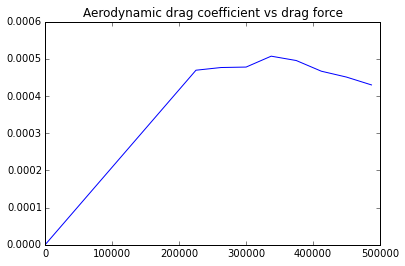# Chapter7-Dimensional Analysis and Simlitude¶

## Ex4-pg288¶

In :
import math
#calculateTest speed in air: and Drag force on prototype
##Diameter of the prototype(in ft):
Dp=1.;
##Speed of towing of prototype(in knots):
Vp=5.;
##Diameter of model(in inches):
Dm=6.;
##Drag for model at test condition(in lbf):
Fm=5.58;
##Density of seawater at 5 C  for prototype(in slug/ft**3):
dp=1.99;
##Kinematic viscosity at 5 C for prototype(in ft**2/sec):
vp=1.69*10**-5;
##Density of air at STP for model(in slug/ft**3):
dm=0.00238;
##Kinematic viscosity of air at STP for model(in ft**2/sec):
vm=1.57*10**-4;
##speed and force##
##Velocity of prototype in ft/sec
Vp1=Vp*6080./3600.
##Reynolds number of prototype:
Rep=Vp1*Dp/vp
##Rep=Rem
##Therefore:
Rem=Rep;
##Velocity of air for wind tunnel(in ft/sec):
Vm=Rem*vm/(Dm/12.)
##Drag force on prototype(in lbf):
Fp=Fm*(dp/dm)*(Vp1/Vm)**2.*(Dp/(Dm/12.))**2
print("RESULTS")
print'%s %.2f %s'%("Test speed in air: ",Vm," ft/sec")
print'%s %.2f %s'%("Drag force on prototype: ",Fp," lbf")

RESULTS
Test speed in air:  156.90  ft/sec
Drag force on prototype:  54.06  lbf


## Ex5-pg294¶

In :
import math
#calculate Drag Force and Power required to pull prototype at 100 kmph
import numpy
%matplotlib inline

import warnings
warnings.filterwarnings('ignore')
##Width of the prototype(in ft):
wp=8.;
##Frontal area of the prototype(in ft**2):
Ap=84.;
##Model Scale:
S=1./16.;
##Density of air(in kg/m**3):
d=1.23;
##Air speed in wind tunnel(in m/sec):
V=numpy.array([18, 21.8, 26, 30.1, 35, 38.5, 40.9, 44.1, 46.7]);
##Drag force(in N):
Fd=numpy.array([3.1, 4.41, 6.09, 7.97, 10.7, 12.9 ,14.7, 16.9, 18.9]);
##Kinematic viscosity(in m**2/sec):
v=1.46*10**-5;
##Density of air(in kg/m**3):
d=1.23;
##Speed of prototype(in km/hr):\
Vp=100.;
##speed force and power##
##Width of the model(in m):
wm=S*wp*0.3048
##Area of model (in m**2):
Am=S**2.*Ap*(0.305)**2
V=numpy.linspace(18.,46.7,num=9)
n=len(V);
Cd=numpy.zeros(n)
Re=numpy.zeros(n)
for i in range (1,n):
Cd[i]=((2.*Fd[i])/d)/((V[i]**2.)/0.0305)
Re[i]=(V[i]*wm)/v
##Reynolds number:

pyplot.plot(Re,Cd);
a=gca()
a.data_bounds=numpy.matrix([[100000,0.4],[500000,0.6]])
pyplot.title('Aerodynamic drag coefficient vs drag force')
##It is seen that drag coefficient becomes constant at CD=0.46above Re=4*10**5 at which speed of air is 40m/s
CDc=0.46;
Va=40;
##Drag force (in N):
FDp=CDc/2*d*(Vp*5/18)**2*Ap*0.305**2
##Power required to pull prototype at 100 kmph(in W)
Pp=FDp*Vp*5/18
print("RESULTS")
print'%s %.2f %s'%("Speed above which Cd is constant: ",Va," m/sec")
print'%s %.2f %s'%("Drag Force: ",FDp/1000," kN")
print'%s %.2f %s'%("Power required to pull prototype at 100 kmph: ",Pp/1000," kWb")

RESULTS
Speed above which Cd is constant:  40.00  m/sec
Drag Force:  1.71  kN
Power required to pull prototype at 100 kmph:  47.38  kWb## Ex6-pg297¶

In :
import math
#calculate Volume flow rate at condition 2 nd and Specific speed at condition 2
##Efficinc of pump:
Effp=0.8;
##Design specific speed(in rpm):
Nscu1=2000.;
##Impeller diameter(in inches):
D1=8.;
##Opertion sped at esign point flow condition(in rpm):
N1=1170.;
##Flow rate at design point flow condition(in gpm):
Q1=300.;
##Density of water (in slug/ft**3):
d1=1.94;
##Acceleration due to gravity(in ft**2/sec):
g=32.2;
##Working speed 2(in rpm):
N2=1750.;
##power and speed##
##The same pump is used for both the conditions.Hence:
D2=D1;
##The same water is used for both the conditions. Hence:
d2=d1;
##Flow rate at condition 2(in gpm):
Q2=Q1*N2/N1*(D2/D1)**3.
H1=(N1*math.sqrt(Q1)/Nscu1)**(4./3.)
H2=H1*(N2/N1)**2.*(D2/D1)**2.
##Pump output power at condition 1(in hp):
P1=d1*g*Q1*H1/7.48/60./550.
##Pump output power at condition 2(in hp):
P2=P1*(d2/d1)*(N2/N1)**3.*(D2/D1)**5.
##Required input power(in hp):
Pin=P2/Effp
##Specific speed at condition 2:
Nscu2=N2*math.sqrt(Q2)/H2**(3./4.)
print("RESULTS\n")
print'%s %.2f %s'%("Volume flow rate at condition 2: ",Q2," gpm\n")
print'%s %.2f %s'%("Head at condition: ",H2," ft\n")
print'%s %.2f %s'%("Pump output power at condition: ",P2," hp\n")
print'%s %.2f %s'%("Required input power: ",Pin," hp\n")
print'%s %.2f %s'%("Specific speed at condition 2: ",Nscu2,"\n")

RESULTS

Volume flow rate at condition 2:  448.72  gpm